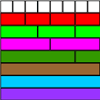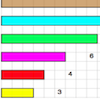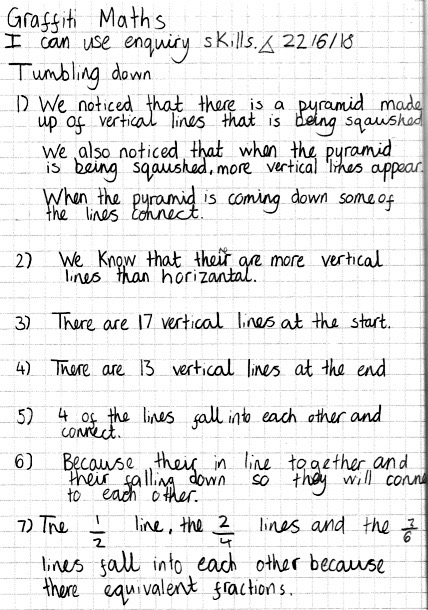#### You may also like### Matching Fractions, Decimals and Percentages

Can you match pairs of fractions, decimals and percentages, and beat your previous scores?### Fractional Wall

Using the picture of the fraction wall, can you find equivalent fractions?### Fraction Lengths

Can you find combinations of strips of paper which equal the length of the black strip? If the length of the black is 1, how could you write the sum of the strips?

# Tumbling Down

##### Age 7 to 11Challenge Level
Thank you to Colneis Junior School in Felixstowe who sent in several solutions.

Part of Harriet's work states:

I could see in the ending image that the first section (coloured orange) and the end section were in line with the first sixth and the last sixth.

Harriet's complete work is here:   Harriet.pdf

Sienna wrote the following:

Q1) What do you notice?
• Every time it shrinks more sections appear.
• The fraction grid starts with 21 sections and travels down to 12 sections.
• If you looked at it from a bird's eye view before it shrinks it would look identical to the end.

Q2) What do you know?
I know that the fraction wall starts with $\frac{1}{6}$, $\frac{1}{5}$ then $\frac{1}{4}$, $\frac{1}{3}$ then $\frac{1}{2}$ then to one whole. Also as you go up the wall the denominators are getting bigger but the fractions are getting smaller.

Q3) How many vertical lines are there at the start?
At the beginning, there are 15 vertical lines excluding the sides but 17 including the sides.

Q4) How many vertical lines are there at the end?
At the end, there are 11 excluding the sides and 13 including the sides.

Q5) Which lines “fall into” others?
Equivalent fractions will fall into other equivalent fractions e.g. $\frac{2}{6}$ will fall into $\frac{1}{3}$ because they are equivalent.

Q6) Explain why. Because they are equivalent.

Q7) Focus on the starting image, how will you add to the pattern?
I tried putting sevenths on the wall and I noticed that it wouldn't submerge to any others because it is not equivalent to any others so when it gets to the end it will be its own line.

My solution for the tumbling down is:
On the wall, you need equal fractions to fall into other equal fractions, but when I added the sevenths it wouldn't fall into any others because it's not equivalent to any other fraction.
If you wanted to add another unequivalent fraction you would have to go up to elevenths because $\frac{1}{9}$ falls into $\frac{1}{3}$ and $\frac{1}{10}$ falls into $\frac{1}{5}$. And also 7 and 11 are prime numbers.

Part of Molly R's work says:

I also noticed throughout the video that different lines were falling on top of different lines.

Her complete work can be viewed here: Molly.pdf

Eden and Martha wrote:

We noticed when we watched the video that the fraction wall begins with seven horizontal lines and ends with two. It begins with 17 vertical lines and ends with 13. The reason for this is because some vertical lines merge into each other. The wall shows a line of symmetry.
We know that there is a line of symmetry at the start and end. At the end, there is one bar of 12 unequal sections. At the start of the video, the fraction wall starts with 15 vertical lines on the inside and two on the outside. At the end, there are 11 vertical lines and two on the outside. We know that lines $\frac{2}{6}$ and $\frac{1}{3}$ merge together to make one line. $\frac{4}{6}$ and $\frac{2}{3}$ merge and lines $\frac{3}{6}$, $\frac{2}{4}$, $\frac{1}{2}$ merge together. We noticed that the lines that merge are equivalent fractions. So that the lines merge, the fraction lines that are at the top fall quicker than the lines towards the bottom.
By adding another bar to the top, your result wouldn't be the same as some fractions (for example sevenths) wouldn't merge as they aren't equivalent to anything.

Here is part of what Vivian wrote:

At the ending point, you can see that all of the lines have joined together. To get the value of the ending parts... I subtracted the fractions from each other.

Vivian's complete work is here:  Vivian .pdf

Aoife wrote:

I noticed that the end image is what you would see if you were looking at it from a bird's eye view. I also noticed that certain lines go into others. There are 17 vertical lines at the beginning and 13 vertical lines at the end.
The lines that fall into each other are equivalent fractions on the wall which is a fraction wall so $\frac{1}{2}$, $\frac{2}{4}$, $\frac{3}{6}$ all go into each other and $\frac{1}{3}$, $\frac{2}{6}$ and $\frac{2}{3}$, $\frac{4}{6}$ fall into each other because the fractions that fall into each other are equivalent.
To continue the wall I would go up to tenths. Now $\frac{1}{2}$, $\frac{2}{4}$, $\frac{3}{6}$, $\frac{4}{8}$, $\frac{5}{10}$ and $\frac{1}{4}$, $\frac{2}{8}$ and $\frac{3}{4}$, $\frac{6}{8}$ and $\frac{1}{3}$, $\frac{2}{6}$, $\frac{3}{9}$ and $\frac{2}{3}$, $\frac{4}{6}$, $\frac{6}{9}$ 'fall into' each other. Nothing would fall into the sevenths because 2x7 = 14 and that is more than 10.

Maisie and Ellie-Rose sent in the following:

We noticed that all the lines go down at the same time and it starts with 12 sections and ends with 12. Also, we found out that some lines are vertical and others are horizontal which makes them overlap and then disappear. We know that the bottom starts with 2, and ends with 12. When the video finishes the shapes are all different. There are 17 vertical lines at the start as there are 15 on the inside and two on the outside. At the end, there are 11 vertical lines within the inside and there are two against the outside.
The fractions of $\frac{2}{6}$ and $\frac{1}{3}$ merge together to make one full line. Then $\frac{4}{6}$ and $\frac{2}{3}$ merge together as well and $\frac{3}{6}$, $\frac{2}{4}$ and $\frac{1}{2}$ this is because they are all equivalent. By adding another bar to the top, your results would not be the same as some fractions, for example, the fraction of sevenths wouldn't merge as they are not equivalent to anything.

Alex's work is shown here:There were some excellent pieces of work here particularly showing good explanations of thoughts and ideas. Well done.